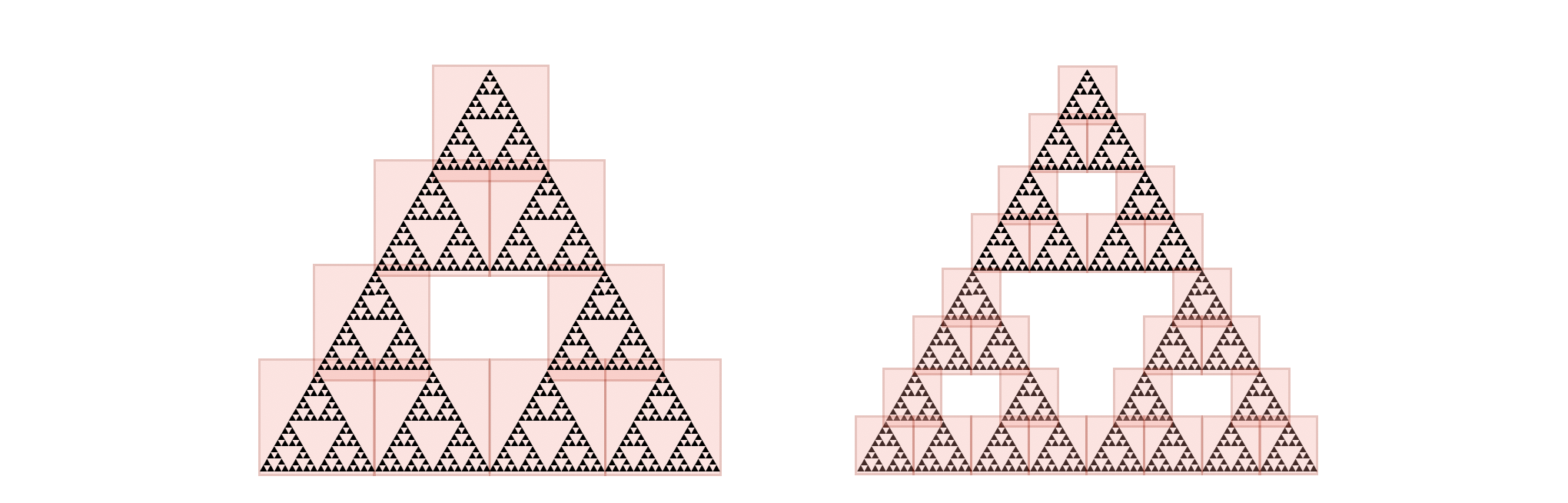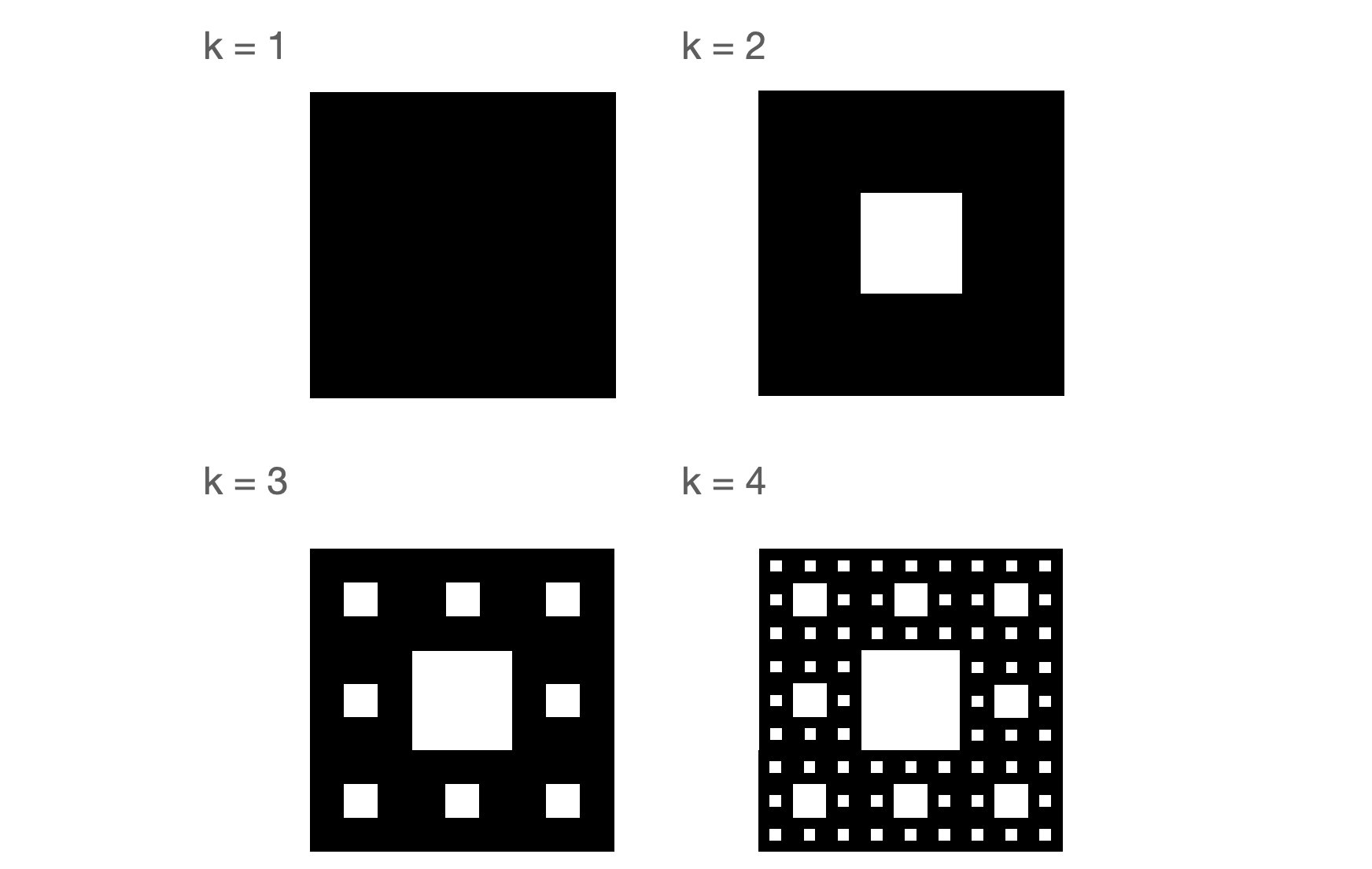## 1.3 But why does it work?

It is a lovely creation. But what properties does it have? How big is its area?

A quick calculation clears up any doubts. In step $$k$$, the triangle consists of $$3^k$$ triangles, each with side $$1/2^k$$, that is, area $$\sqrt{3}/2^{2k+2}$$. The total area of what remains after step $$k$$ is $$(3/4)^k \cdot \sqrt{3}/4$$. In the limit, we get:

$\lim_{k \rightarrow \infty} (3/4)^k \cdot \sqrt{3}/4 = 0.$

The Sierpiński triangle is, therefore, so hollow that it has an area equal to 0. And how long is its perimeter? In step $$k$$, the perimeter increases by $$3^{k-1}$$ triangles, each with side of length $$(1/2)^k$$, that is, perimeter of $$3 \cdot (1/2)^k$$. The total perimeter length in a step $$k$$ is:

$\sum_{i=1}^k 3^{k-1} \cdot 3 \cdot (1/2)^k = \sum_{i=1}^k (3/2)^k.$

The elements of this sum grow to infinity, so the whole edge of the triangle consequently explodes to infinity:

$\lim_{k \rightarrow \infty}\sum_{i=1}^k (3/2)^k = \infty.$

What is this figure? It is bounded because it fits in a triangle with side 1 but has an infinite perimeter with zero area. What dimension is it from?

Probably by this point, some readers are asking themselves what fractals are. Are they just some strange images? And if so, how do you characterize them?

One of the more frequently repeated definitions of fractals is the one given by Mandelbrot, which I will paraphrase:

Definition (fraktal). A fractal is a set whose fractal dimension is higher than the topological dimension.

As will become clear in a moment, often the fractal dimension doesn’t need to be an integer, hence the name fractal, from the French fractus and the Latin frangěre – to break, fray, fractional.

But what is this topological and fractal dimension?

### 1.3.1 Topological dimension

When we think of Euclidean spaces, the dimension is defined by the number of orthogonal directions spanning a given space. Therefore, a point has dimension 0, a line has dimension 1, a plane has dimension 2, and we all experience a three-dimensional space. We can describe specific objects in higher-dimensional spaces, there are no limitations. A mathematician can also talk excitedly about the function space, which is infinitely dimensional.

Similarly, we can think about the dimensions of sets. Intuitively, the dimension of a set will be the number of orthogonal segments that fit in that set. However, one should be careful. Everything seems to be correct; a point has dimension 0, a line segment has dimension 1, a square has dimension 2, a cube has dimension 3, and so on. However, what to do with a circle? No line segment fits in it. But after all, it should have a dimension greater than 0 because it is a kind of line segment with the ends glued together. So ideally, it should also have dimension $$1$$.

Therefore, the issue is more complicated. Mathematicians have been thinking about it for a long time, and the formal definition of the dimension for sets is relatively young. The first inductive definition was proposed less than 100 years ago independently by Paul Urson and Karl Menger (1922-1923). Today we have at least a few different definitions of dimension. Those interested will find them, along with many interesting facts, in the article A few words about dimension [Nowa11] (Polish: Kilka słów o wymiarze).

For further analysis, we will keep the intuitive definition – with the number of orthogonal vectors that can be embedded in the analyzed set.

### 1.3.2 Minkowski dimension, or fractal box-counting dimension

There are also several definitions of fractal dimension but probably the most popular is Minkowski’s definition, often called box-counting dimension. It is determined for geometric objects embedded in ordinary $$p$$-dimensional spaces (we will practically limit ourselves only to $$p=2$$). To determine it, we need to count how many $$p$$-dimensional boxes of side $$\varepsilon$$ can cover the object of interest.

Mandelbrot, when writing about fractal dimension, referred to the definition of the Hausdorff dimension, which is a generalization of the Minkowski dimension. In Hausdorff’s definition, instead of equal-sized boxes, we can cover the analyzed object with any family of sets with diameters converging to 0. However, this margin is too small to accurately represent and explain the Hausdorff dimension, so let’s stay with the box dimension.

If $$N(\varepsilon)$$ is the minimum number of boxes of side $$\varepsilon$$ covering an object $$F$$, then the box-counting dimension $$d_F$$ of this object is:

$d_F = \lim_{\varepsilon \rightarrow 0} \frac{\log N(\varepsilon)}{\log 1/\varepsilon}.$

In general, this limit may not exist. In such situations, the upper and lower limits of this sequence can be considered separately. But for the objects presented in this book, the so-defined limit always converges to something. Moreover, it does not matter what size of boxes we choose. However, I show examples for boxes that `tightly’ cover parts of the figure of interest to make calculations easier.

Let’s assume for now that we are interested in boxes of side $$\varepsilon = 2^{-k}$$. If we want to cover a square of side $$1$$ with such boxes, we need at least $$N(\varepsilon) = 2^k * 2^k = 2^{2k}$$ of them. Therefore, the box-counting dimension of the square is:

$d_F = \lim_{k \rightarrow \infty} \frac{\log 2^{2k}}{\log 1/2^{-k}} = 2.$

What about the Sierpiński triangle? Here we only need $$N(\varepsilon) = 3^k$$ boxes of side $$2^{-k}$$, so the box dimension for this triangle is:

$d_F = \lim_{k \rightarrow \infty} \frac{\log 3^{k}}{\log 1/2^{-k}} = \frac{\log 3}{\log 2} = 1,5849\ldots$

Figure 4 illustrates what an example of covering a Sierpiński triangle with boxes of sides 1/4 and 1/8 looks like.Rysunek 4: Covering the Sierpiński triangle with boxes of sides 1/4 (left panel) and 1/8 (right panel)

What is the dimension of Cantor dust? This time, we have an object in one-dimensional space, so we will cover it with line segments. Suppose we have line segments of length $$\varepsilon = 3^{-k}$$. To cover the entire Cantor dust, we need $$N(\varepsilon) = 2^k$$ such segments; that is, the box dimension for Cantor dust is:

$d_F = \lim_{k \rightarrow \infty} \frac{\log 2^{k}}{\log 1/3^{-k}} = \frac{\log 2}{\log 3} = 0,6309\ldots$

### 1.3.3 Sierpinski carpet

We already know what fractals are characterized by. It is time to get to know more representatives of this amazing family. Another well-known example is the Sierpiński carpet.

Recipe for Sierpiński carpet construction.

1. Take a square of any size.
2. Divide this square into nine squares.
3. Remove the inside of the middle square; you will get eight squares with a side 1/3 of the initial square.
4. For each of the resulting eight squares, continue the division by returning to step 2.

For an illustration of the four consecutive steps of the biting algorithm, see [Figure 5].Figure 5: The first four iterations in the construction of the Sierpiński carpet.

What is the box dimension of this object? Let’s take boxes of side $$\varepsilon = 3^{-k}$$. To cover the carpet, we need $$N(\varepsilon) = 8^k$$ boxes of such size. So the box dimension of this carpet is:

$d_F = \lim_{k \rightarrow \infty} \frac{\log 8^{k}}{\log 1/3^{-k}} = \frac{\log 8}{\log 3} = 1,8927...$

### Bibliography

[Nowa11]
Nowak, Sławomir: Kilka słów o wymiarze. In: Delta Bd. 11 (2011)